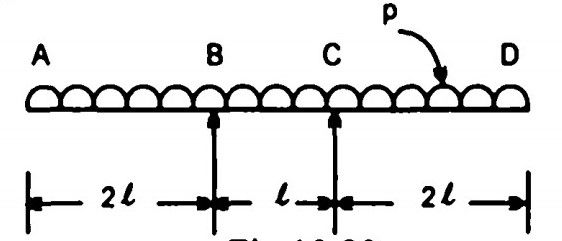top of pageSearch

# Strength of Materials(321-340)

Updated: Apr 29, 2020

321.A timber beam of rectangular section 100 mm x 50 mm is simply supported at the ends, has a 30 mm x 10 mm steel strip securely fixed to the top surface as shown in Fig30 mm

322. The 'Euler' load for a column is 1000 kN and crushing load is 1500 kN. The

600 kN

323. Fig. shows a retaining wall of base width B and height H. The sp. gravity of the material of construction is S. Further AB = BC = CD = B/3When the depth of storage increases from 0 to Hi, the resultant force will move

from B to C

324. A column section as indicated in Fig is loaded with a concentrated load at

a point 'P' so as to produce maximum bending stress due to eccentricities about

xx axis and yy axis as 5 t/m² and 8 t/m² respectively.If the direct stress due to loading is 15 t/m² (compressive), then the intensity of

resultant stress at the corner 'B' of the column section is

12 t/m² (compressive)

325.Two similar round bars A and B are each 30 cm long as shown in Fig.The ratio of the energies stored by the bars A and B,

3/2

326.A. Maximum principal stress - 18.0 B. Minimum principal stress - -8.0 C. Maximum shear stress - 13.0 D. Normal stress on the plane of maximum shear stress - 5.0

327

A. Impulse - Time effect of a force B. Torsion - Modulus of rigidity C. Plane of loading - Shear centre D. Instantaneous centre of rotation - Plane motion

328. A truss ABC, carries two horizontal and two vertical loads, as shown in FigThe horizontal and vertical components of the reaction at A will be

329.In the cantilever truss shown in Fig. , the reaction at A is30 t

330.331.The deflection at the free end of a cantilever subjected to a couple M at its

free end and having a uniform flexural rigidity EI throughout its length 'L' is

equal to

ML²/2EI

332. In Fig., the maximum bending moment at the fixed end of the cantilever caused by the UDL is M 4% of M333. The shear force diagram for a simply

supported beam of span T is given in FigThe maximum bending moment is

Wl

334.

List 1 - List2

A. Clapeyron - Theorem of three moments B. Hardycross - Moment distribution method C. Lame - Thick cylinders D. Euler - Method for determining crippling load on a column

335. A propped cantilever of span T carries a uniformly distributed load of 'w' per unit run over its entire span. The value of prop reaction to keep the beam horizontal is (3/8)wl

336. ABCD is a beam of length 5/ which is supported at B and C (having supported

length BC = 1) and having two equal overhangs AB and CD of length 21 each. It carries a u.d.l. of intensity p per unit length throughout the beam as shown in

Fig.The points of contraflexure will occur

nowhere in the beam

337.The structure shown in Fig. is stable if

x = 2 y

338. Two simply supported beams B and B have spans l and 2l respectively. Beam B has a cross section of 1 x 1 units and beam B has a cross section of 2 x 2 units. These beams are subjected to concentrated load W each at the centre of their spans. The ratio of the maximum flexural stress in these beams is 4

339. In a particular material, if the modulus of rigidity is equal to the bulk modulus, then the Poisson's ratio will be 1/8

340. Consider the following statements : When two planes at right angles are

subjected to direct stresses, the Y axis which denotes shear stress, will pass

through the centre of the Mohr's circle when the direct stresses are

1. equal in magnitude

2. of opposite signs.